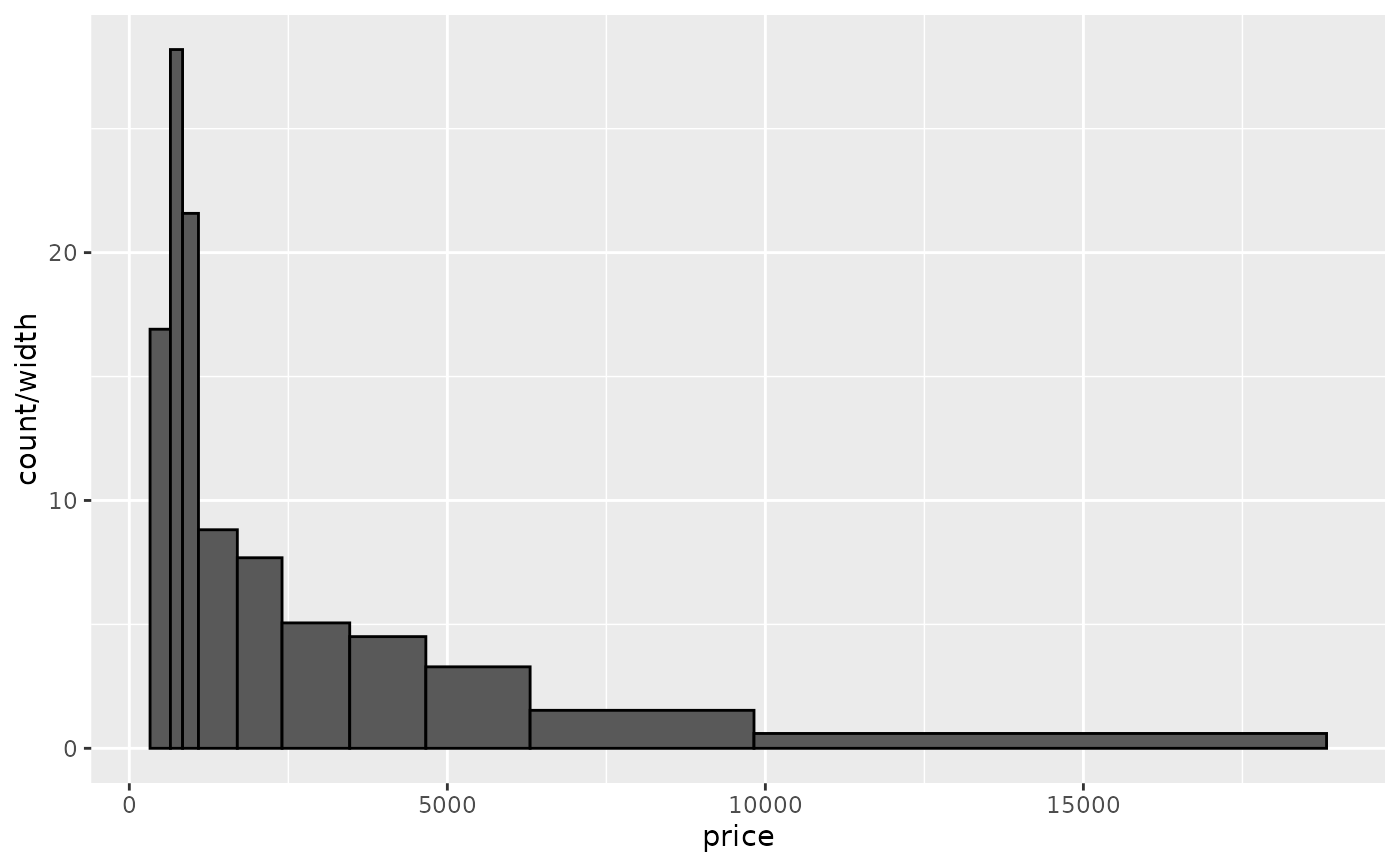Visualise the distribution of a single continuous variable by dividing the x axis into bins and counting the number of observations in each bin. Histograms (geom_histogram()) display the counts with bars; frequency polygons (geom_freqpoly()) display the counts with lines. Frequency polygons are more suitable when you want to compare the distribution across the levels of a categorical variable.

## Usage

geom_freqpoly(
mapping = NULL,
data = NULL,
stat = "bin",
position = "identity",
...,
na.rm = FALSE,
show.legend = NA,
inherit.aes = TRUE
)

geom_histogram(
mapping = NULL,
data = NULL,
stat = "bin",
position = "stack",
...,
binwidth = NULL,
bins = NULL,
na.rm = FALSE,
orientation = NA,
show.legend = NA,
inherit.aes = TRUE
)

stat_bin(
mapping = NULL,
data = NULL,
geom = "bar",
position = "stack",
...,
binwidth = NULL,
bins = NULL,
center = NULL,
boundary = NULL,
breaks = NULL,
closed = c("right", "left"),
na.rm = FALSE,
orientation = NA,
show.legend = NA,
inherit.aes = TRUE
)

## Arguments

mapping

Set of aesthetic mappings created by aes(). If specified and inherit.aes = TRUE (the default), it is combined with the default mapping at the top level of the plot. You must supply mapping if there is no plot mapping.

data

The data to be displayed in this layer. There are three options:

If NULL, the default, the data is inherited from the plot data as specified in the call to ggplot().

A data.frame, or other object, will override the plot data. All objects will be fortified to produce a data frame. See fortify() for which variables will be created.

A function will be called with a single argument, the plot data. The return value must be a data.frame, and will be used as the layer data. A function can be created from a formula (e.g. ~ head(.x, 10)).

position

Position adjustment, either as a string naming the adjustment (e.g. "jitter" to use position_jitter), or the result of a call to a position adjustment function. Use the latter if you need to change the settings of the adjustment.

...

Other arguments passed on to layer(). These are often aesthetics, used to set an aesthetic to a fixed value, like colour = "red" or size = 3. They may also be parameters to the paired geom/stat.

na.rm

If FALSE, the default, missing values are removed with a warning. If TRUE, missing values are silently removed.

show.legend

logical. Should this layer be included in the legends? NA, the default, includes if any aesthetics are mapped. FALSE never includes, and TRUE always includes. It can also be a named logical vector to finely select the aesthetics to display.

inherit.aes

If FALSE, overrides the default aesthetics, rather than combining with them. This is most useful for helper functions that define both data and aesthetics and shouldn't inherit behaviour from the default plot specification, e.g. borders().

binwidth

The width of the bins. Can be specified as a numeric value or as a function that calculates width from unscaled x. Here, "unscaled x" refers to the original x values in the data, before application of any scale transformation. When specifying a function along with a grouping structure, the function will be called once per group. The default is to use the number of bins in bins, covering the range of the data. You should always override this value, exploring multiple widths to find the best to illustrate the stories in your data.

The bin width of a date variable is the number of days in each time; the bin width of a time variable is the number of seconds.

bins

Number of bins. Overridden by binwidth. Defaults to 30.

orientation

The orientation of the layer. The default (NA) automatically determines the orientation from the aesthetic mapping. In the rare event that this fails it can be given explicitly by setting orientation to either "x" or "y". See the Orientation section for more detail.

geom, stat

Use to override the default connection between geom_histogram()/geom_freqpoly() and stat_bin().

center, boundary

bin position specifiers. Only one, center or boundary, may be specified for a single plot. center specifies the center of one of the bins. boundary specifies the boundary between two bins. Note that if either is above or below the range of the data, things will be shifted by the appropriate integer multiple of binwidth. For example, to center on integers use binwidth = 1 and center = 0, even if 0 is outside the range of the data. Alternatively, this same alignment can be specified with binwidth = 1 and boundary = 0.5, even if 0.5 is outside the range of the data.

breaks

Alternatively, you can supply a numeric vector giving the bin boundaries. Overrides binwidth, bins, center, and boundary.

closed

One of "right" or "left" indicating whether right or left edges of bins are included in the bin.

If TRUE, adds empty bins at either end of x. This ensures frequency polygons touch 0. Defaults to FALSE.

## Details

stat_bin() is suitable only for continuous x data. If your x data is discrete, you probably want to use stat_count().

By default, the underlying computation (stat_bin()) uses 30 bins; this is not a good default, but the idea is to get you experimenting with different number of bins. You can also experiment modifying the binwidth with center or boundary arguments. binwidth overrides bins so you should do one change at a time. You may need to look at a few options to uncover the full story behind your data.

In addition to geom_histogram(), you can create a histogram plot by using scale_x_binned() with geom_bar(). This method by default plots tick marks in between each bar.

## Orientation

This geom treats each axis differently and, thus, can thus have two orientations. Often the orientation is easy to deduce from a combination of the given mappings and the types of positional scales in use. Thus, ggplot2 will by default try to guess which orientation the layer should have. Under rare circumstances, the orientation is ambiguous and guessing may fail. In that case the orientation can be specified directly using the orientation parameter, which can be either "x" or "y". The value gives the axis that the geom should run along, "x" being the default orientation you would expect for the geom.

## Aesthetics

geom_histogram() uses the same aesthetics as geom_bar(); geom_freqpoly() uses the same aesthetics as geom_line().

## Computed variables

These are calculated by the 'stat' part of layers and can be accessed with delayed evaluation.

• after_stat(count)
number of points in bin.

• after_stat(density)
density of points in bin, scaled to integrate to 1.

• after_stat(ncount)
count, scaled to a maximum of 1.

• after_stat(ndensity)
density, scaled to a maximum of 1.

• after_stat(width)
widths of bins.

## Dropped variables

weight

After binning, weights of individual data points (if supplied) are no longer available.

stat_count(), which counts the number of cases at each x position, without binning. It is suitable for both discrete and continuous x data, whereas stat_bin() is suitable only for continuous x data.

## Examples

ggplot(diamonds, aes(carat)) +
geom_histogram()
#> stat_bin() using bins = 30. Pick better value with binwidth.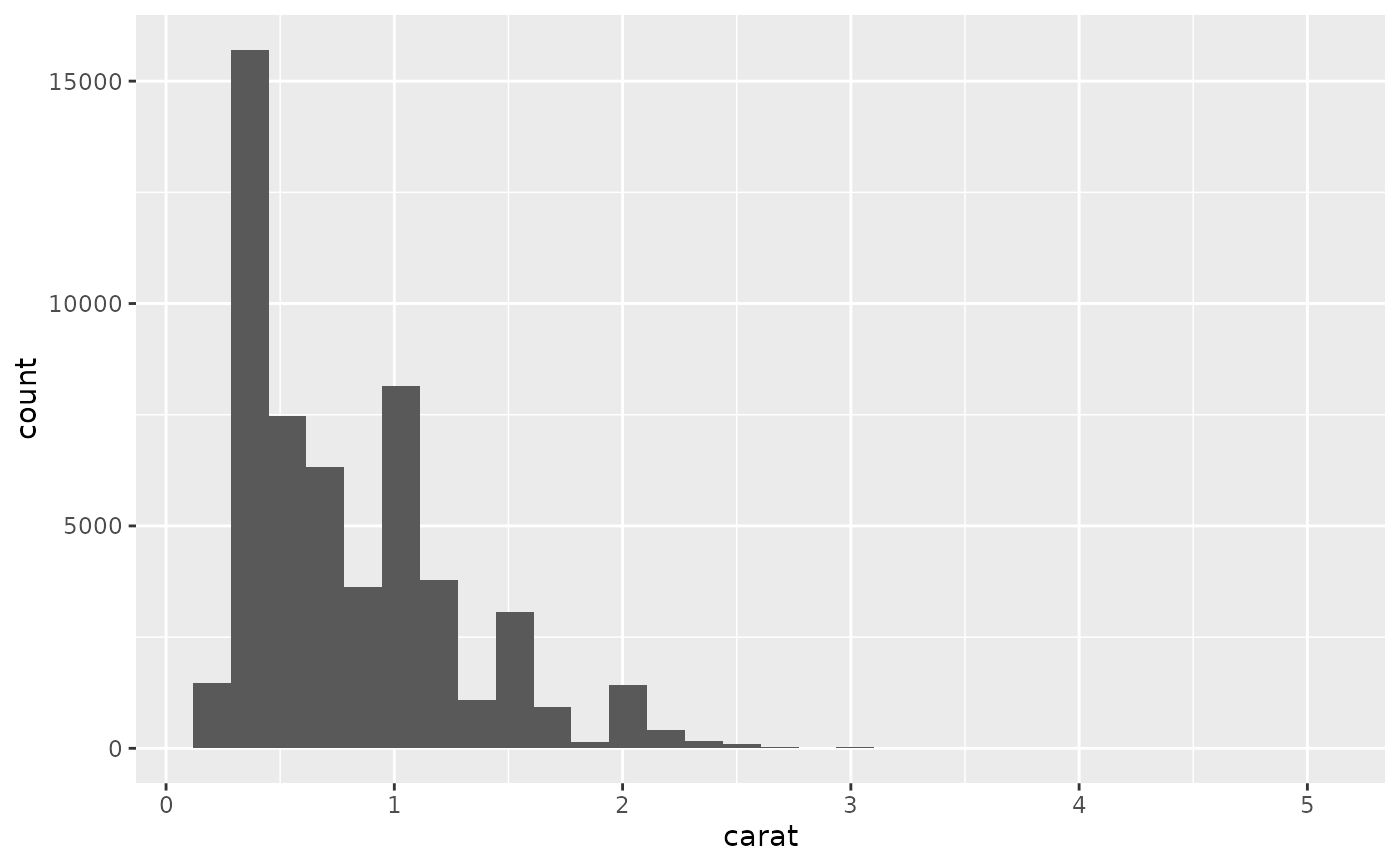ggplot(diamonds, aes(carat)) +
geom_histogram(binwidth = 0.01)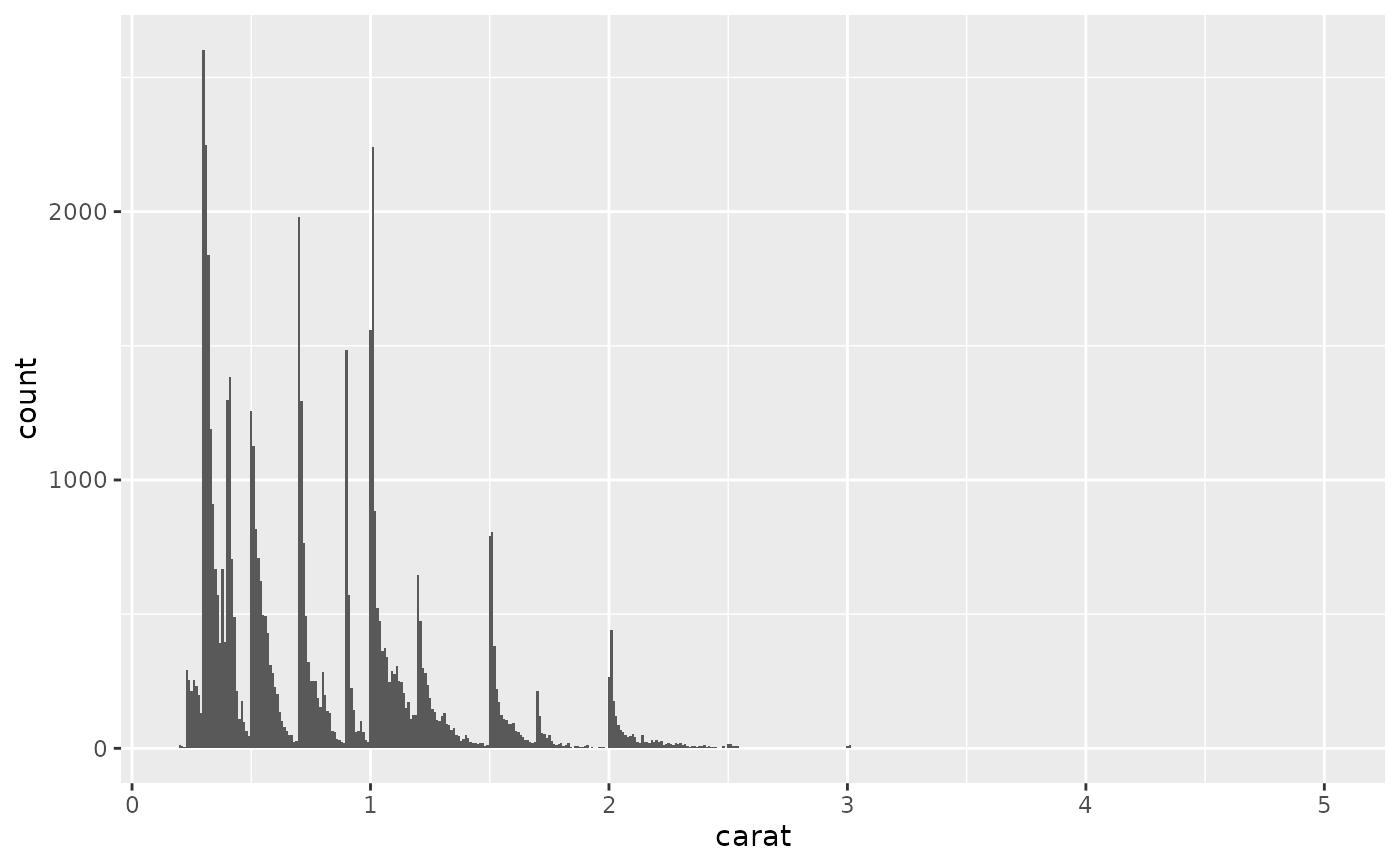ggplot(diamonds, aes(carat)) +
geom_histogram(bins = 200)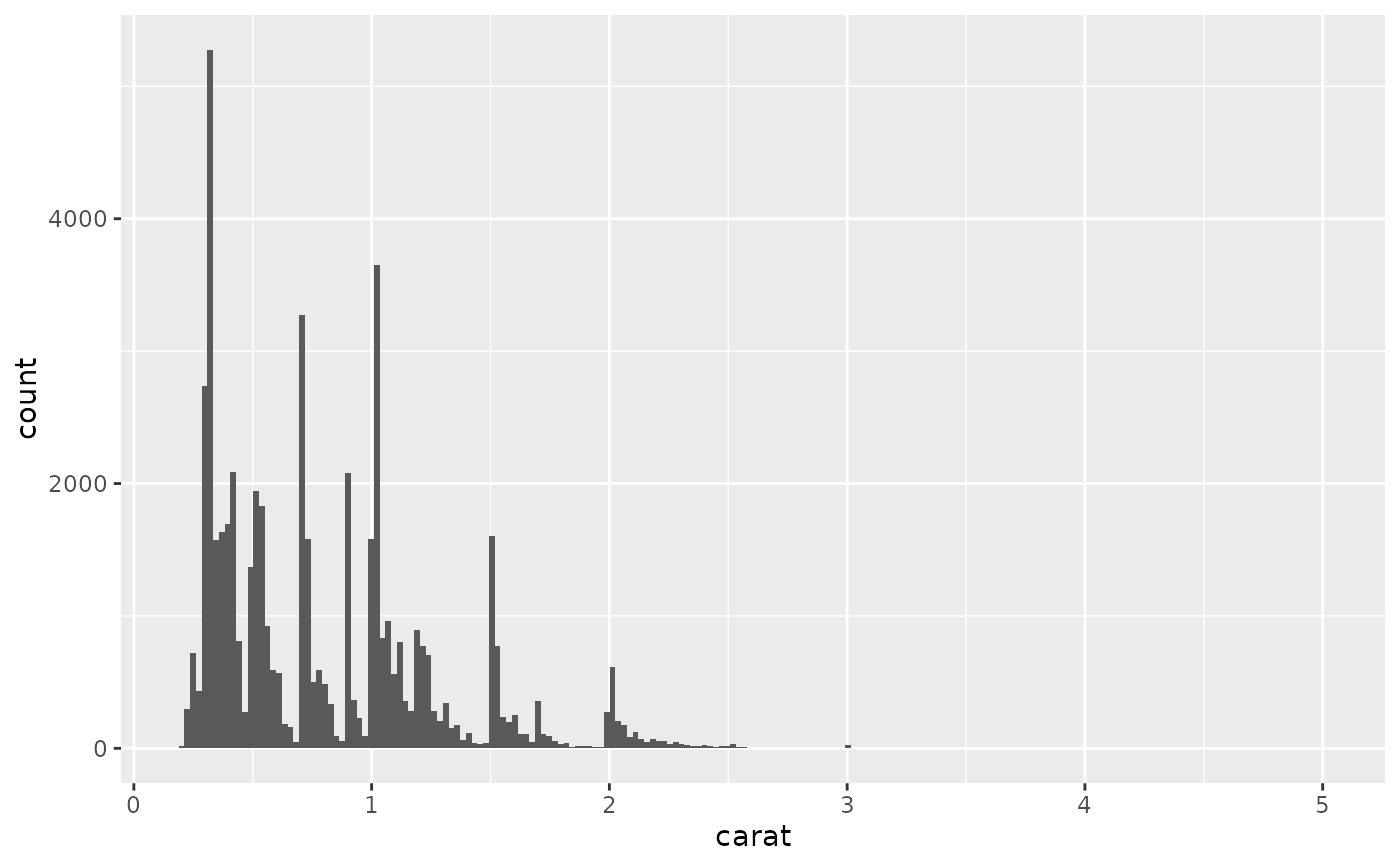# Map values to y to flip the orientation
ggplot(diamonds, aes(y = carat)) +
geom_histogram()
#> stat_bin() using bins = 30. Pick better value with binwidth.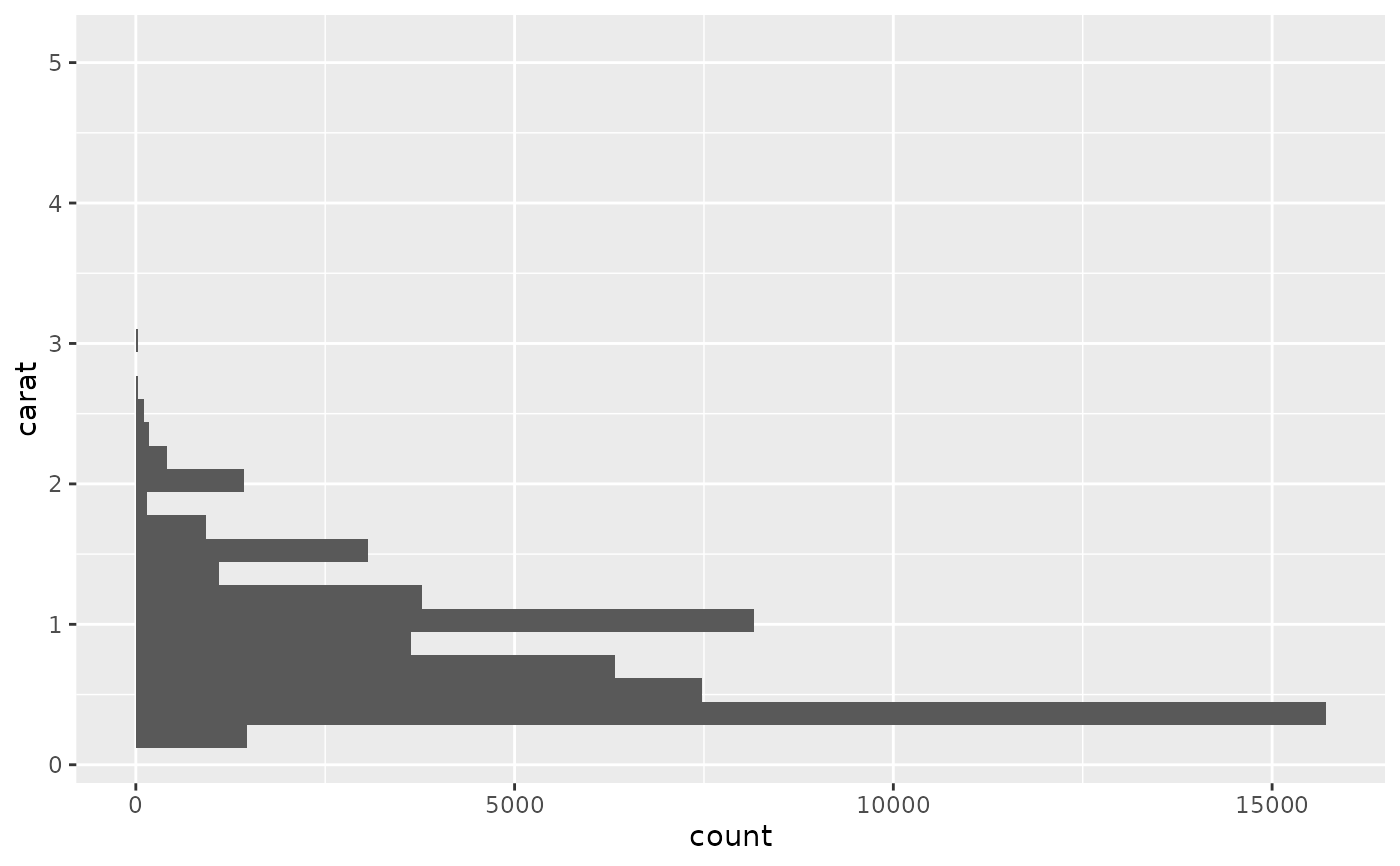# For histograms with tick marks between each bin, use geom_bar() with
# scale_x_binned().
ggplot(diamonds, aes(carat)) +
geom_bar() +
scale_x_binned()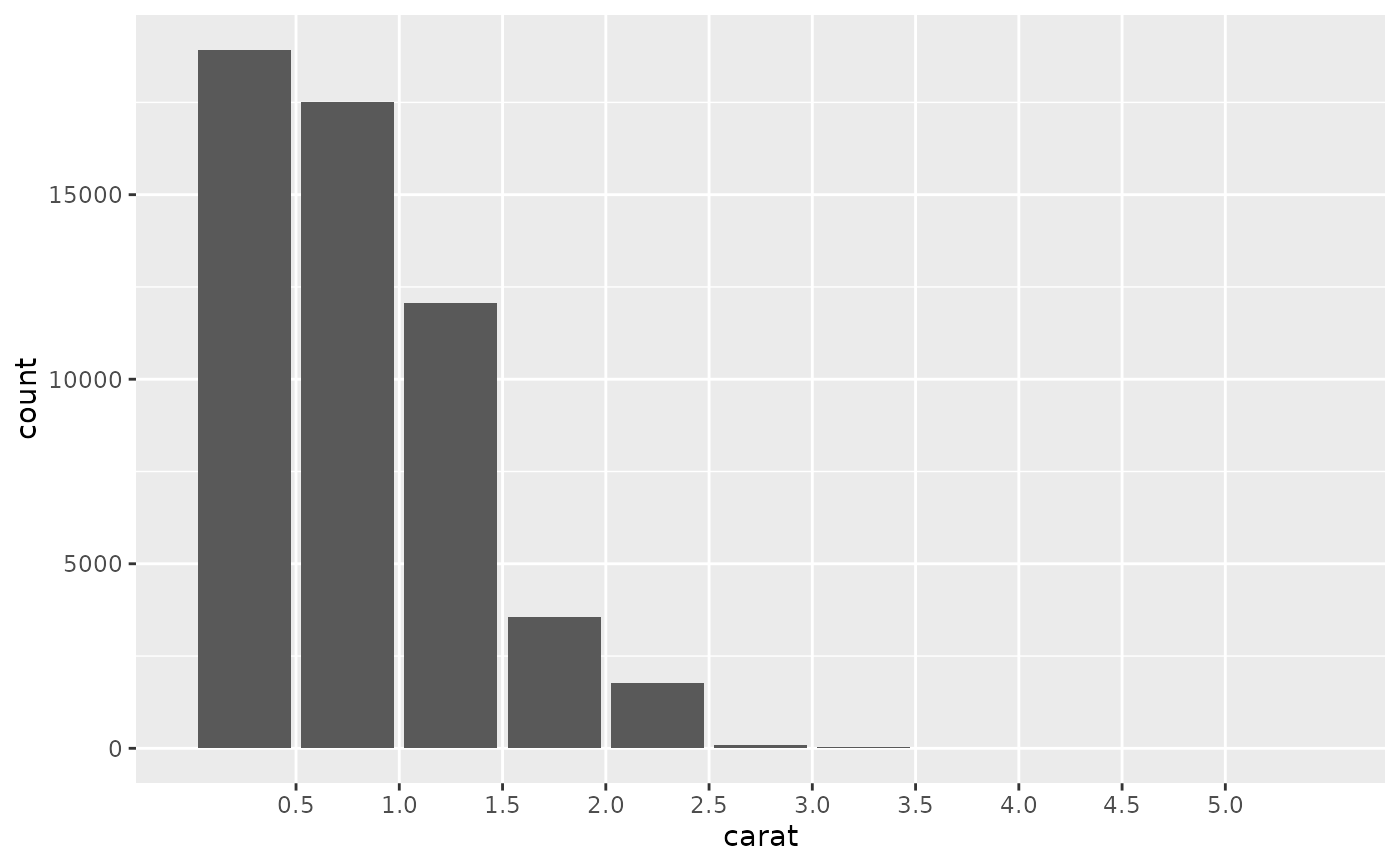# Rather than stacking histograms, it's easier to compare frequency
# polygons
ggplot(diamonds, aes(price, fill = cut)) +
geom_histogram(binwidth = 500)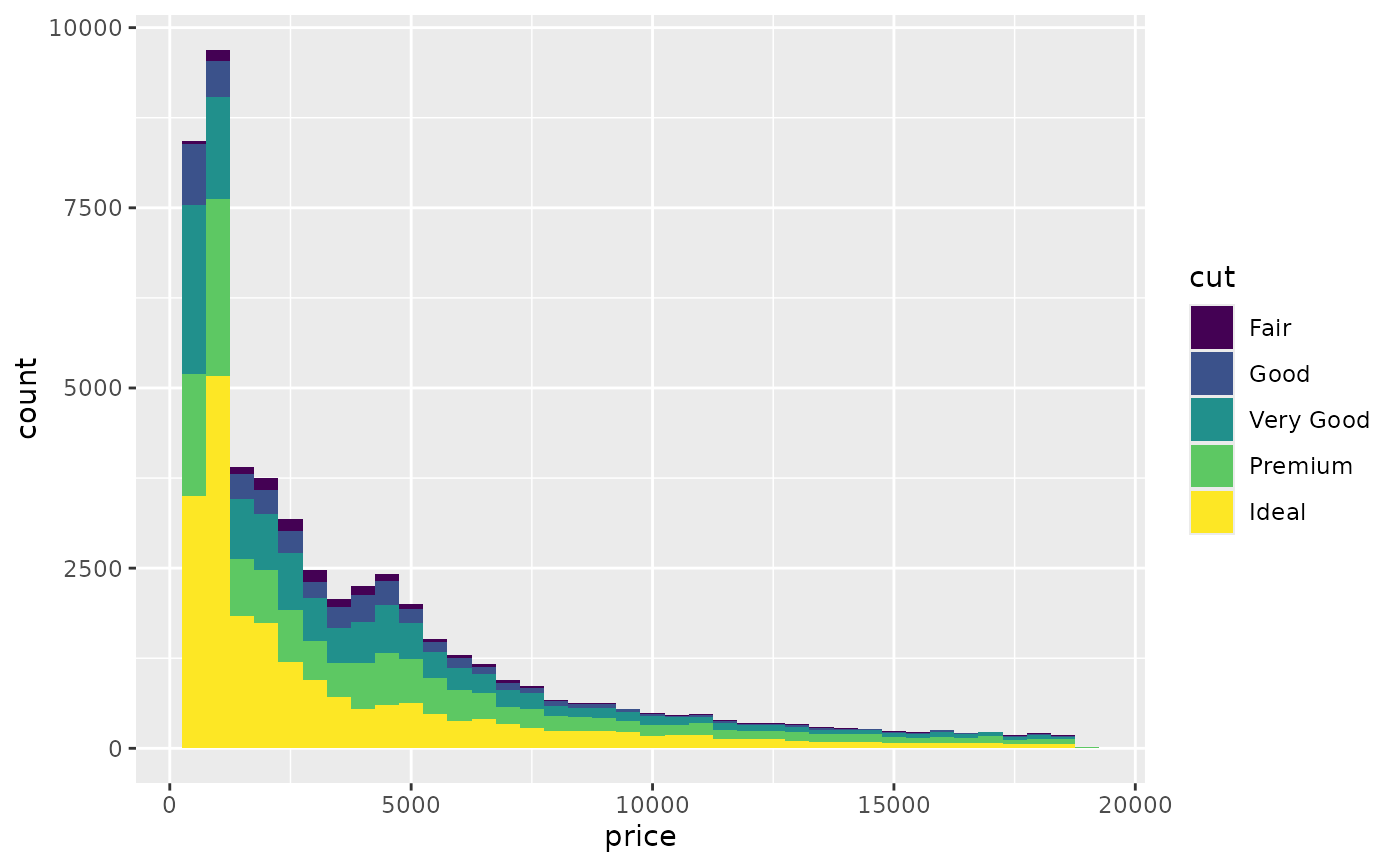ggplot(diamonds, aes(price, colour = cut)) +
geom_freqpoly(binwidth = 500)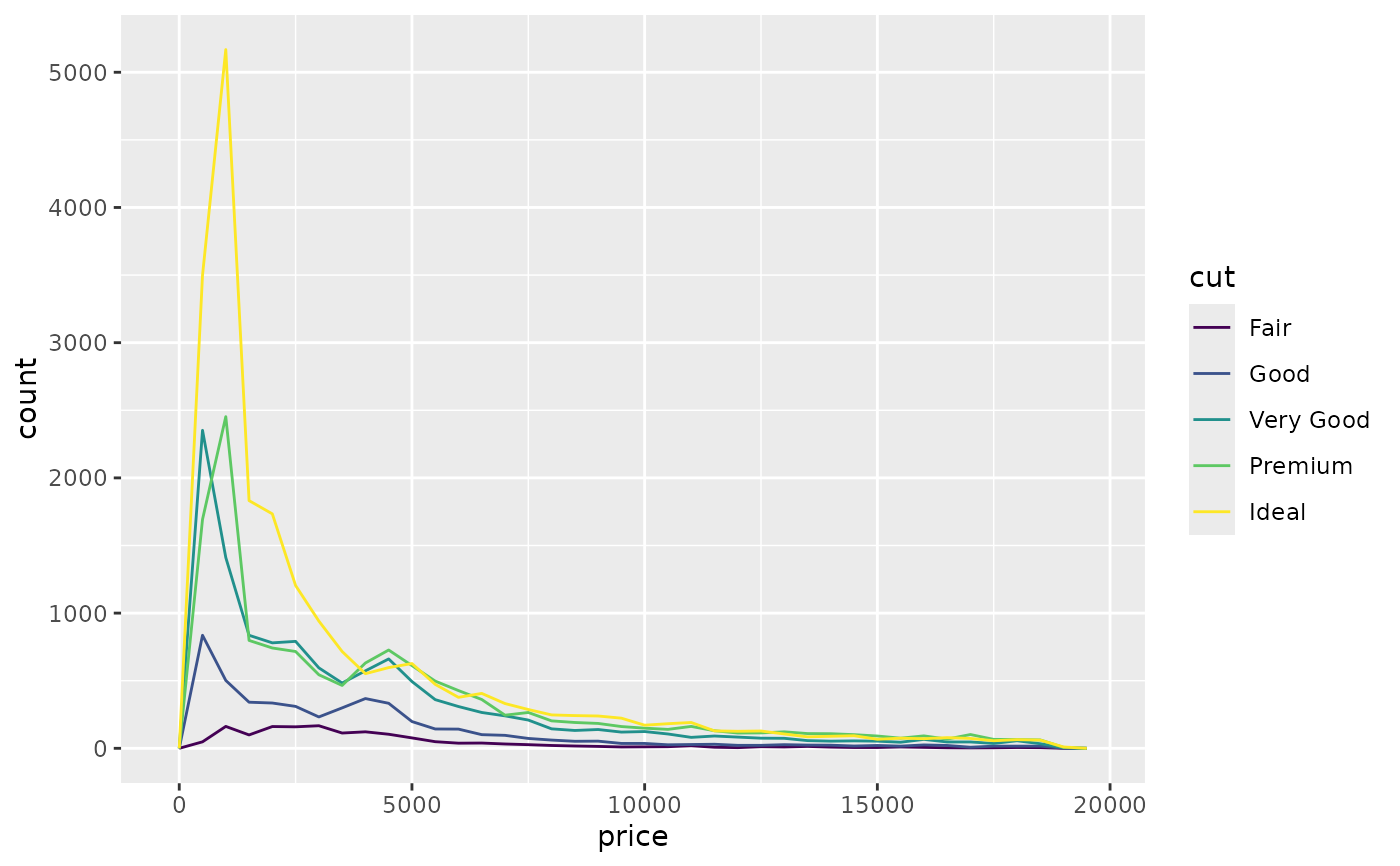# To make it easier to compare distributions with very different counts,
# put density on the y axis instead of the default count
ggplot(diamonds, aes(price, after_stat(density), colour = cut)) +
geom_freqpoly(binwidth = 500)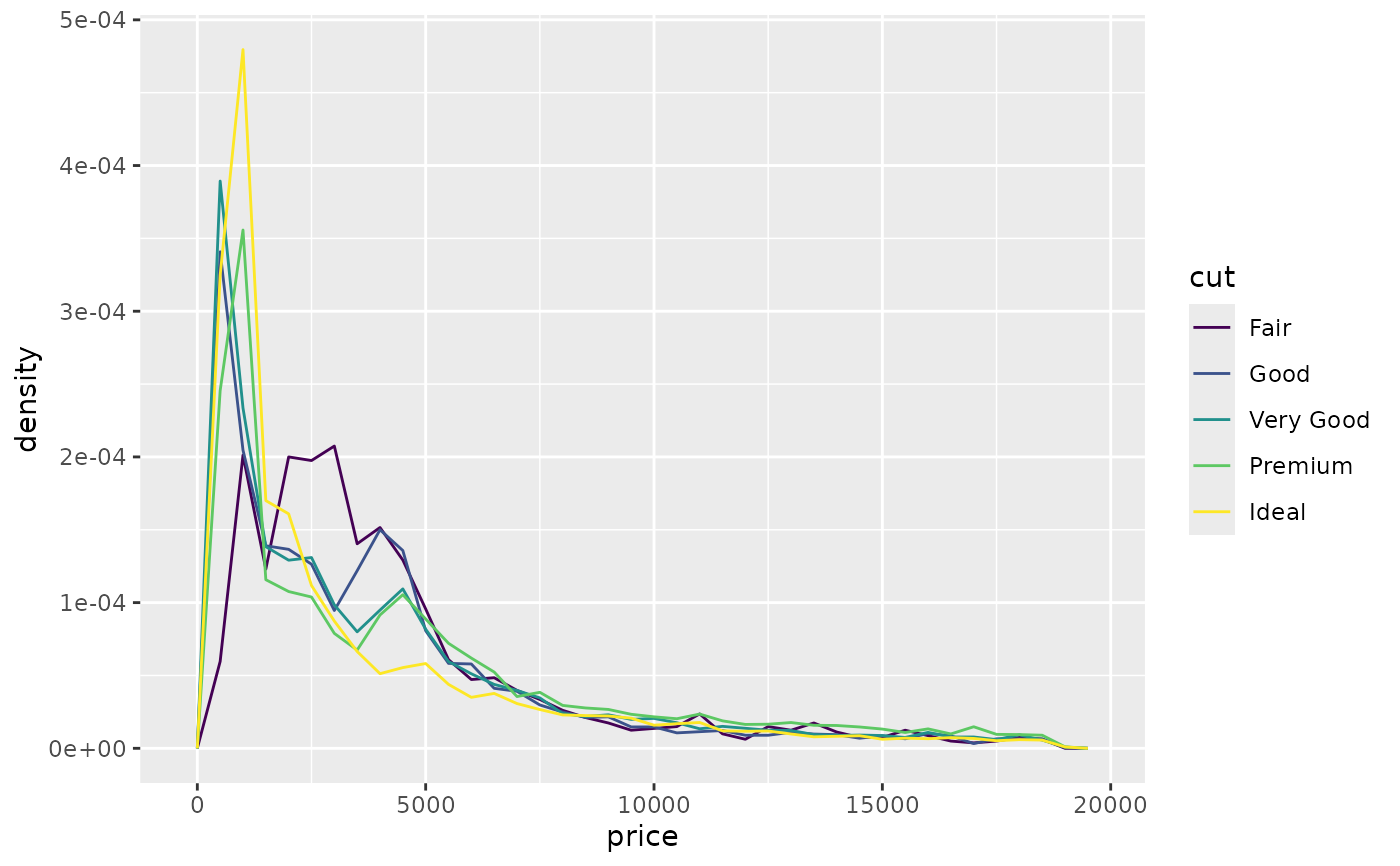if (require("ggplot2movies")) {
# Often we don't want the height of the bar to represent the
# count of observations, but the sum of some other variable.
# For example, the following plot shows the number of movies
# in each rating.
m <- ggplot(movies, aes(rating))
m + geom_histogram(binwidth = 0.1)

# If, however, we want to see the number of votes cast in each
# category, we need to weight by the votes variable
m +
geom_histogram(aes(weight = votes), binwidth = 0.1) +

# For transformed scales, binwidth applies to the transformed data.
# The bins have constant width on the transformed scale.
m +
geom_histogram() +
scale_x_log10()
m +
geom_histogram(binwidth = 0.05) +
scale_x_log10()

# For transformed coordinate systems, the binwidth applies to the
# raw data. The bins have constant width on the original scale.

# Using log scales does not work here, because the first
# bar is anchored at zero, and so when transformed becomes negative
# infinity. This is not a problem when transforming the scales, because
# no observations have 0 ratings.
m +
geom_histogram(boundary = 0) +
coord_trans(x = "log10")
# Use boundary = 0, to make sure we don't take sqrt of negative values
m +
geom_histogram(boundary = 0) +
coord_trans(x = "sqrt")

# You can also transform the y axis.  Remember that the base of the bars
# has value 0, so log transformations are not appropriate
m <- ggplot(movies, aes(x = rating))
m +
geom_histogram(binwidth = 0.5) +
scale_y_sqrt()
}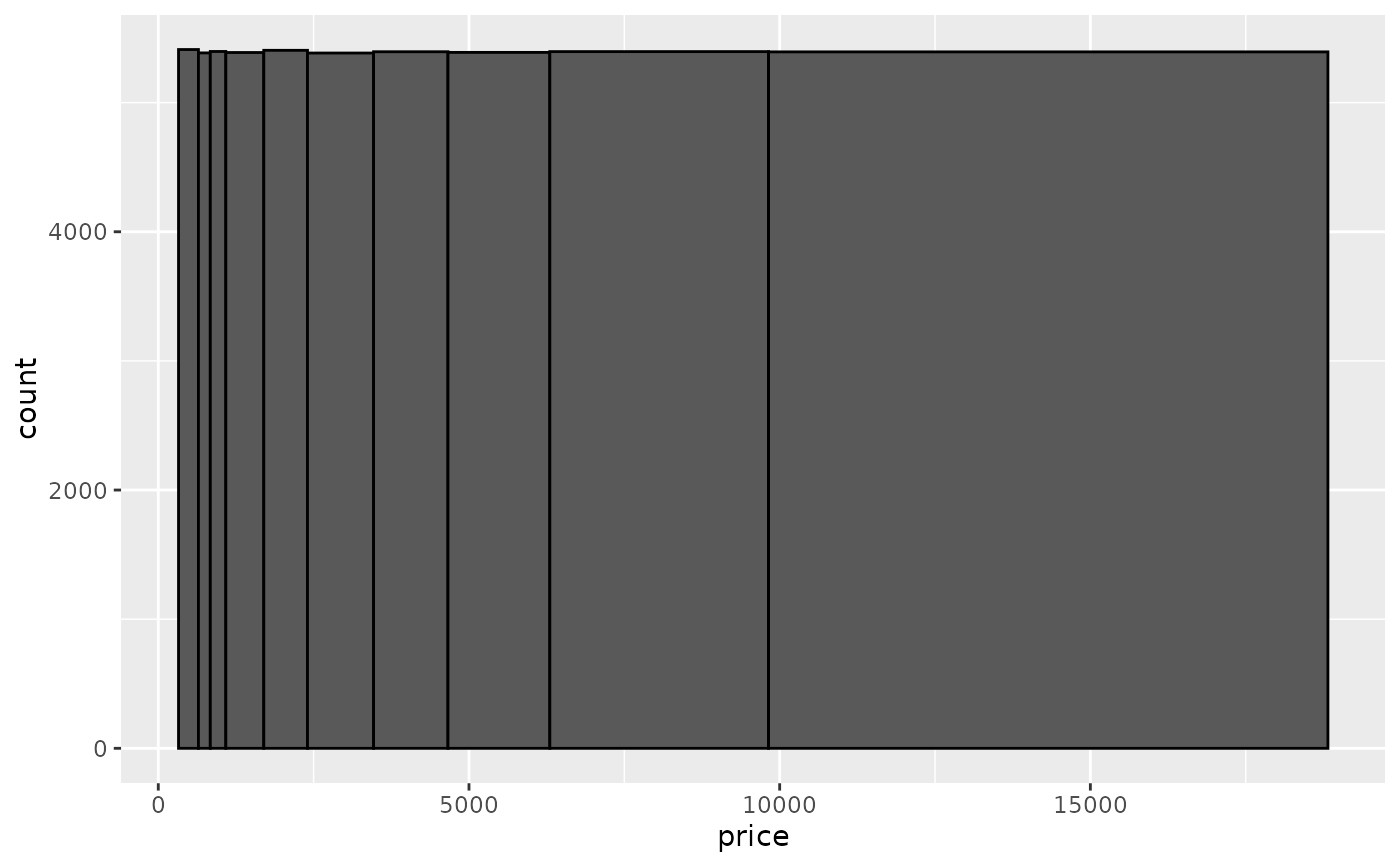# You can specify a function for calculating binwidth, which is
# particularly useful when faceting along variables with
# different ranges because the function will be called once per facet
ggplot(economics_long, aes(value)) +
facet_wrap(~variable, scales = 'free_x') +
geom_histogram(binwidth = function(x) 2 * IQR(x) / (length(x)^(1/3)))